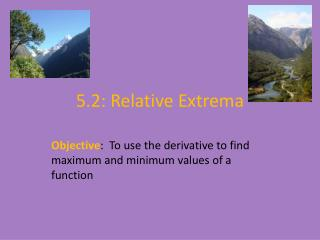Download Presentation5.2: Relative Extrema

# 5.2: Relative Extrema - PowerPoint PPT PresentationDownload Presentation## 5.2: Relative Extrema

- - - - - - - - - - - - - - - - - - - - - - - - - - - E N D - - - - - - - - - - - - - - - - - - - - - - - - - - -
##### Presentation Transcript

1. 5.2: Relative Extrema Objective: To use the derivative to find maximum and minimum values of a function

2. Discuss what is happening in this graph. Are there any maximum or minimum points?

3. RELATIVE MAXIMUM OR MINIMUM • Let c be a number in the domain of f. • f(c) is a relative (or local) maximum if on the open interval (a,b)f(x) < f(c) for all x in (a,b) (f(c) is the largest value) • f(c) is a relative (or local) minimum if on the open interval (a,b)f(x) > f(c) for all x in (a,b) (f(c) is the smallest value) • A function has a relative (or local) extremum (plural extrema) if at c there is either a local max or min

4. Relative Extrema at Endpoints • If c is an endpoint of the domain of f, consider what is happening to the left or right of endpoint: • If c is a left-hand endpoint: • Relative max if f is decreasing to the right of c • Relative min if f is increasing to the right of c • If c is a right-hand endpoint: • Relative max if f is increasing to the left • Relative min if f is decreasing to the left

5. Identify the x-values of all the points where the graph has relative extrema:

6. If a function has a relative extremum at c, then c is a critical number or c is an endpoint of the domain…This is a big deal!!!! • This does not mean that all critical numbers are relative extrema →

7. FIRST DERIVATIVE TEST!!! • Find critical numbers, c • Test the sign of f’(x) to the left and the right of critical numbers • f(c) is a relative max if: • f’(x) > 0 to the left of c • f’(x) < 0 to the right of c • f(c) is a relative min if: • f’(x) < 0 to the left of c • f’(x) > 0 to the right of c • For endpoints, it depends if it is a left or right-hand endpoint • If no sign change, no relative extreme values at c

8. Find the x value of all points where the function has relative extrema. Find the value(s) of any relative extrema.

9. ALGEBRA HAT→

10. EXPONENTIAL FUNCTION HAT→

11. ex HAT→